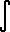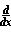Home    |    Teacher    |    Parents    |    Glossary    |    About UsEmail this page to a friendResources· Cool Tools · Formulas & Tables · References · Test Preparation · Study Tips · Wonders of MathSearchProof: Integral ex (Math | Calculus | Integrals | Table Of | ex)

 Discussion ofex dx = ex + C.
1. Proof
Since we know the derivative:ex = ex,
we can use the Fundamental Theorem of calculus:ex dx =(ex) dx = ex + C
Q.E.D.

See also the proof thatex = exPROOF